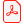# Konferensartikel

## Deriving the Beam Equation using the Minimum Total Potential Energy Principle and Solving the Equation Numerically

Magnus Komperød
Technological Analyses Centre, Nexans Norway AS, Norway

Ladda ner artikelhttp://dx.doi.org/10.3384/ecp1815365

Linköping Electronic Conference Proceedings 153:10, s. 65-72

Visa mer +

ISBN: 978-91-7685-494-5

ISSN: 1650-3686 (tryckt), 1650-3740 (online)

### Abstract

The beam equation describes the deflection of a beam subject to point loads and / or distributed loads, while being supported at both ends. The beam equation is commonly derived in the scientific literature using force- and moment balances, which lead to a boundary value problem. The present paper derives the beam equation using the minimum total potential energy principle and solves the optimization problem numerically. The motivation behind this work is to ease future extensions of the beam equation into larger deflections and nonlinear materials. These future extensions are necessary to model subsea power cables and umbilicals during bending stiffness tests which is the author’s final goal.

### Nyckelord

Beam Equation, Bending Stiffness Test, Minimum Total Potential Energy Principle, Numerical Analysis, Subsea Power Cable, Umbilical

### Referenser

Inga referenser tillgängliga